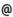## Babes-Bolyai University of Cluj-Napoca Faculty of Mathematics and Computer Science Study Cycle: Graduate SUBJECT

 Code Subject MMG0005 Geometry 4 (Geometry of Differentiable Varieties)
 Section Semester Hours: C+S+L Category Type Mathematics 4 2+1+0 speciality compulsory
 Teaching Staff in Charge
 Assoc.Prof. PINTEA Cornel, Ph.D.,  cpinteamath.ubbcluj.roProf. VARGA Csaba Gyorgy, Ph.D.,  csvargacs.ubbcluj.ro
 Aims The course is a natural continuation of the course \$Curves and Surfaces\$ from spring semester in the first year and studies the main geometrical objects associated to a differential manifold. The tutorials complets, by examples, applications, execices and problems, the theoretical material given at the course.
 Content 1.The n-dimensional Euclidean space from algebraic and topological point of view. Differentiable mappings. The inverse function theorem. The rank theorem. Regular points and critical points. 2. Differential manifolds. Examples. Differentiable mappings between manifolds. The tangent space and the tangent map. The cotangent space and the cotangent map. Differentiable submanifolds. Immersions, submersions, embedings. 3. The tangent fiber bundle and its sections. Vector fields on a manifold. Global and local flows. Integrability and completeness. The Lie algebra of vector fields. 4. Fiber bundles and linear connections.
 References 1. AUSLANDER, L. - MACKENZIE, R.E.: Introduction to Differentiable Manifolds, McGraw- Hill, 1963 2. CONLON, L.: Differentiable Manifolds, Birkhauser, 1993 3. KOSINSKI, A.: Differential Manifolds, Academic Press, 1993 4. LEE, J.M.: Smooth Manifolds, Springer, 2001 5. MATSUSHIMA, Y.: Differentiable Manifolds, Marcel Dekker, 1972 6. PINTEA, C, Geometrie. Geometrie Diferentiala. Geometrie Riemanianna. Grupuri si Algebre Lie, Presa Universitara Clujeana, 2006.
 Assessment Exam (70%)+Tutorial activities (30%).
 Links: Syllabus for all subjects Romanian version for this subject Rtf format for this subject